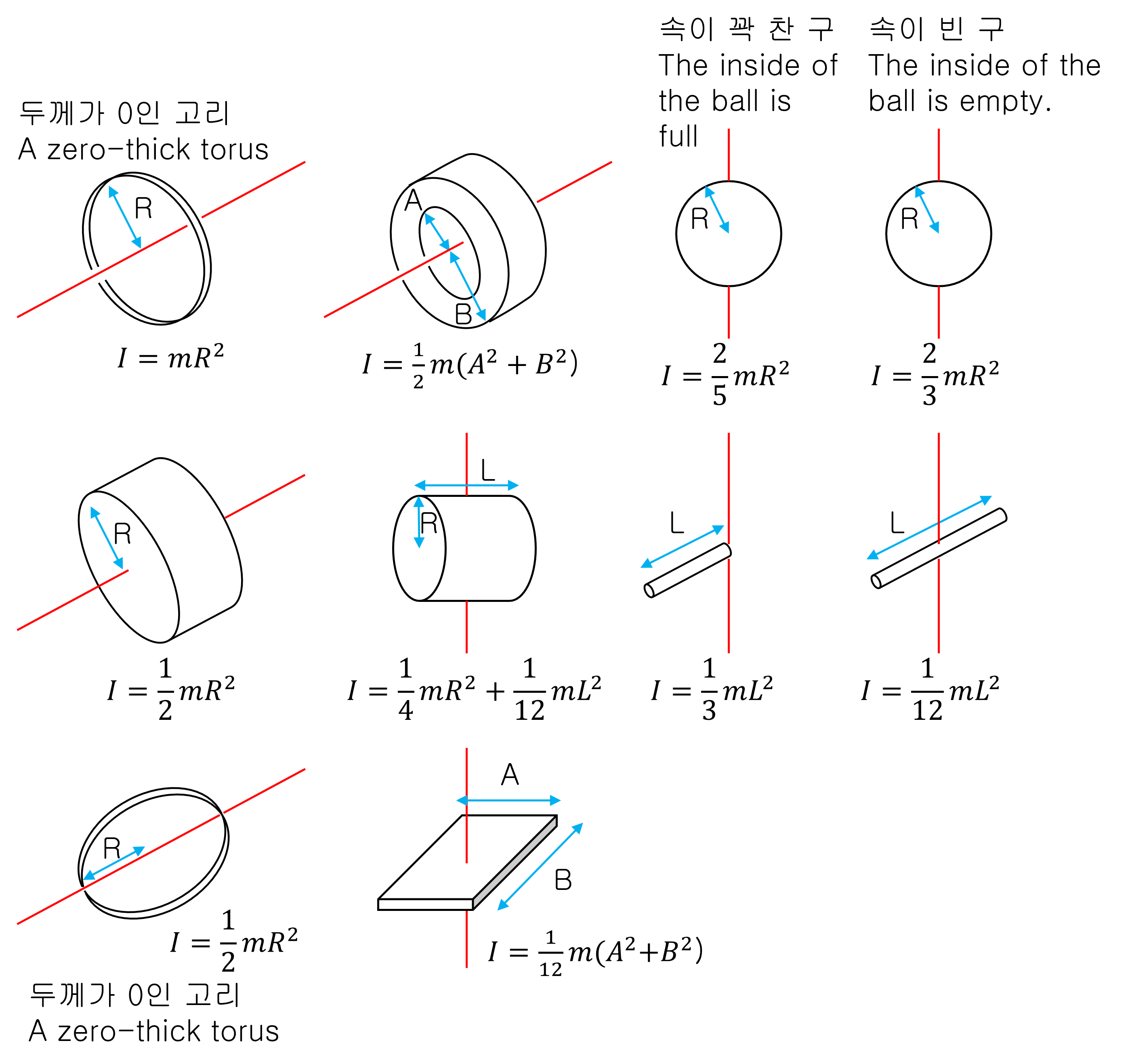# Moment of Inertia (3D)

It’s a tube. The radius of the tube is outside: inside = 0.75: 1. There is no rolling friction.
It’s a tube. The radius of the tube is outside: inside = 0.5: 1. There is no rolling friction.
It’s a tube. The radius of the tube is outside: inside = 0.25: 1. There is no rolling friction.
It’s a cylinder. There is no rolling friction.
It’s a brick. There is no sliding friction.

## Why does a rolling object go down slower than an object that slides down?

In conclusion, the rolling object wasted some energy to rotate. In contrast, an object that slides without rotation can convert given potential energy into 100% kinetic energy.

Assuming there is no sliding friction, an object’s total energy is divided into energy for movement and rotational energy.

Total energy = translational kinetic energy + rotational kinetic energy

$K\, =\, \frac { 1 }{ 2 } m{ v }^{ 2 }\, +\, \frac { 1 }{ 2 } I{ \omega }^{ 2 }$

$$m$$: Mass of object(kg)
$$v$$: Speed of the object’s center of mass(m/s)
$$I$$: Moment of inertia of an object(kg.m²)
$$w$$: Angular velocity of an object(rad/s)

If the radius is $$R$$, the angular velocity $$\omega = \frac { v }{ R }$$ for non-slip pure rolling motion. Therefore, the total kinetic energy K can be expressed as shown bwlow:

$K\, =\, \frac { 1 }{ 2 } (\frac { I }{ { R }^{ 2 } } +m){ v }^{ 2 }$

The source of energy that causes this object to move is the potential energy on the oblique plane.
If x is the length the object rolls down, then the potential energy of the oblique plane is shown below:

$E\, =\, mgx\cdot sin(\theta )$

The energy $$E$$ supplied and the energy $$K$$ used are the same.

$mgx\cdot sin(\theta )\, =\, \frac { 1 }{ 2 } (\frac { I }{ { R }^{ 2 } } +m){ v }^{ 2 }$

We can simplify the expression by substituting exercise relation ‘$${ v }^{ 2 }\, =\, 2ax$$’,

$\begin{split} mgx\cdot sin(\theta )\, &=\, \frac { 1 }{ 2 } (\frac { I }{ { R }^{ 2 } } +m)\cdot 2ax \\ mg\cdot sin(\theta )\, &=\, (\frac { I }{ { R }^{ 2 } } +m)\cdot a \end{split}$

After all, We can express the acceleration $$a$$ of the translational motion:

$\therefore a\, =\, \frac { g }{ (\frac { I }{ m{ R }^{ 2 } } +1) } sin(\theta )$

Substituting the moment of inertia $$I$$ of a rolling object in the above equation, we can find the rolling object’s rolling acceleration. Thus, if an object rolls down in any way, it will go slower than if it simply slipped without friction.
Another interesting fact is that if an object’s shape is the same, the acceleration is the same regardless of its size. For example, a sphere of the same shape will have the same acceleration, even if the spheres are different sizes.

## Moment of inertia ‘$$I$$’

If ‘inertia’ is a property of maintaining motion in a linear motion. And the moment of inertia is a physical quantity that indicates the degree to which rotational motion is maintained in rotational motion.
In other words, a rotating object will continue to rotate if no external force is applied.

Inertia is proportional to the mass of an object, whereas the moment of inertia is influenced by the shape of the object and the mass.
Here are the moments of inertia for different shaped objects.